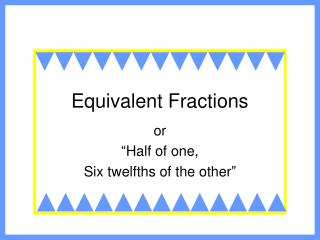DownloadDownload PresentationEquivalent Fractions

# Equivalent Fractions

Télécharger la présentation## Equivalent Fractions

- - - - - - - - - - - - - - - - - - - - - - - - - - - E N D - - - - - - - - - - - - - - - - - - - - - - - - - - -
##### Presentation Transcript

1. Equivalent Fractions or “Half of one, Six twelfths of the other”

2. What is a fraction? • A fraction is part of a whole that is made up of equal parts.

3. And here’s the person who ate it. 1 8 • And here is 1/8 of the pizza. 7 8 • Here is 7/8 of the pizza because there are 7 out of 8 slices left. Part of a whole what? • Well, it could be part of a whole object, like the proverbial pizza . . . • Here is a whole pizza.

4. Was there something else? • Yes, it could also be part of a whole group of items. • Here is a group of cookies. 1 5 • We could say 1/5 of these cookies are chocolate chip. We could also say that 4/5 are Oreos. 4 5 3 4 • Oops, let’s make that 3/4 are Oreos.

5. So, what are equivalent fractions? • Well, sometimes an object or a group of items can be divided differently, and fractions can name the same amount.

6. Say, what? • Okay, try this. Let’s take a rectangle and divide it into 8 parts. • Now, if we color 2 parts, we say that 2/8 of the rectangle are shaded. 2 8

7. I’m with you. What’s next? • Now, let’s take that same rectangle and divide it into 16 parts. • If we color 4 parts, we say that 4/1 of the rectangle are shaded. 4 16

8. So that means? • So that means 2/8 is equivalent to 4/1 . 2 8 4 16 • And we write it this way: = 2 8 4 16

9. Give us another example. • Okay, how about: 3 9 1 3 3 9 1 3 =

10. How do you find equivalent fractions? • You can multiply (or divide), but you must multiply (or divide) both the numerator AND denominator by the same number. 1 4 3 12 x3 = x3 2 5 4 10 x2 = x2

11. What about dividing? • Here’s how. 4 20 1 5 ÷ 4 = ÷ 4 4 14 2 7 ÷ 2 = ÷2

12. What if you’re not sure? • Here is how you can check to see if two fractions are equivalent. • You can “cross-multiply.” 5 x 2 = 10 1 5 2 10 = 1 x 10 = 10 • Since both products are the same, these two fractions are equivalent.

13. How do you know if they’re not equivalent? • Here is an example. You still “cross-multiply.” 3 x 4 = 12 2 3 4 5 = 2 x 5 = 10 • Since both products are NOT the same, these two fractions are NOT equivalent.

14. Now what? • Let’s do some practice activities in the computer lab. • Here’s an activity we will do.

15. Here’s what! • Open MY COMPUTER.

16. Then… • Go to the X DRIVE.

17. Navigate to our class folder (Class Files  5th Grade  Postman).

18. Navigate to our class folder (Class Files  5th Grade  Postman).

19. Navigate to our class folder (Class Files  5th Grade  Postman).

20. Navigate to our class folder (Class Files  5th Grade  Postman).

21. It will look something like this.

22. Go to VIEW and add a header for your name. Save on the N DRIVE. Save every few minutes.

23. 1 4 2 8 • Highlight all the cells you need to shade for the first fraction on the first table. =

24. 1 4 2 8 • Then, shade the second fraction on the second table. =

25. 1 4 3 • Continue until you have completed all the examples. On some you will need to complete one or more of the fractions. Just click where the number goes, and type. =

26. On the last one, you need to create your own tables. Go to TABLE on the menu bar. Drag down to INSERT. Drag across to TABLE.

27. Choose the number of columns and rows you will need.

28. Save and print when you are done. • Are there any questions? • I’ll give you the written directions in the lab. • Let’s go!